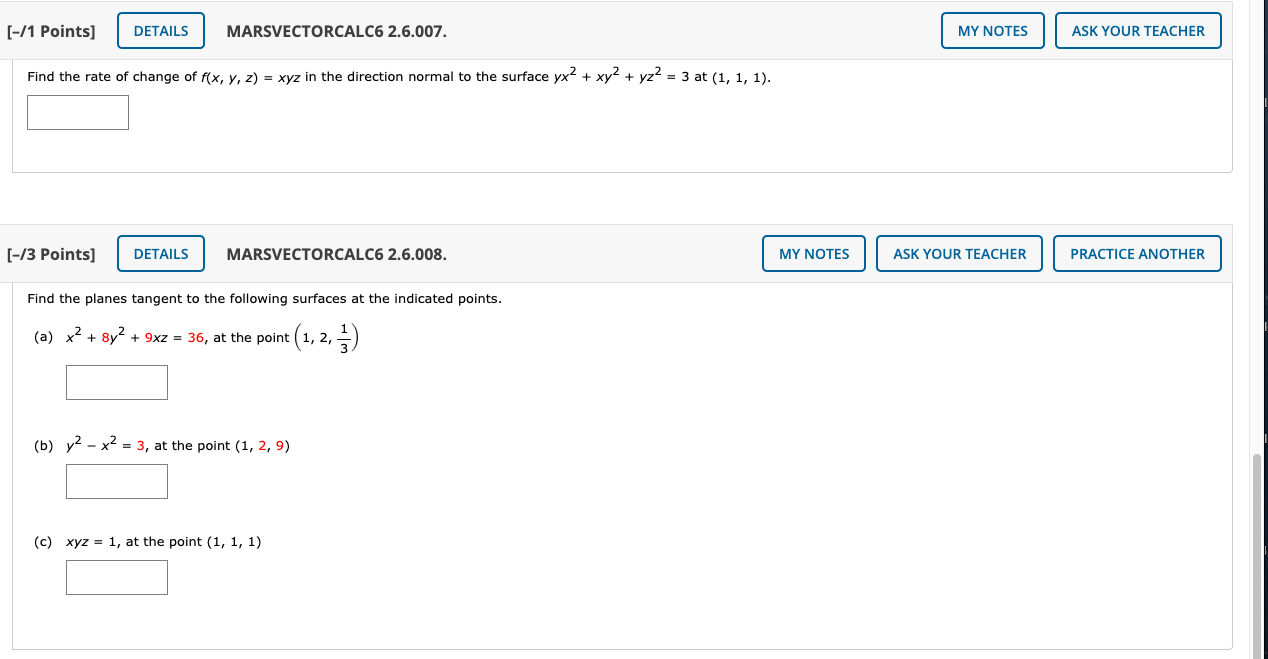# Question (-/1 Points] DETAILS MARSVECTORCALC6 2.6.007. MY NOTES ASK YOUR TEACHER Find the rate of change of f(x, y, z) = xyz in the direction normal to the surface yx2 + xy2 + yz= 3 at (1, 1, 1). [-/3 Points] DETAILS MARSVECTORCALC6 2.6.008. MY NOTES ASK YOUR TEACHER PRACTICE ANOTHER Find the planes tangent to the following surfaces at the indicated points. (a) x2 + 8y2 + 9xz = 36, at the point t(1, 2, 5) 1 (b) y2 - x2 = 3, at the point (1, 2, 9) (c) xyz = 1, at the point (1, 1, 1)